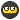## Recommended Posts

not sure if tthis helps

http://www.edu-observatory.org/gps/gps_accuracy.htmlhttp://users.erols.com/dlwilson/gpswtavg.htm

Edited by Old Sailor

How is DOP (GDOP, HDOP, PDOP, TDOP, and VDOP) calculated?

Alternatively, can someone point me to a good reference: book or website?

I am looking for the equation with elements.

Twinstars,

There is an article, "GPS Error Analysis", by Brad Parkinson, which shows in detail how to calculate GDOP. The article is in the book, "Global Positioning System: Theory and Applications: Volume 1". The calculations you want are illustrated on page 475.

It's a bit cumbersome to fully reproduce here, but a simplified example is that you take the 4 satellites that give you the best geometry. Using the Azimuth and Elevation of each, you set up a 4x4 matrix as follows:

cos(E1)sin(Az1) cos(E1)cos(Az1) sin(E1) 1

cos(E2)sin(Az2) cos(E2)cos(Az2) sin(E2) 1

cos(E3)sin(Az3) cos(E3)cos(Az3) sin(E3) 1

cos(E4)sin(Az4) cos(E4)cos(Az4) sin(E4) 1

If you multiply that matrix by it's transpose, then invert that resultant matrix, you end up with the "DOP matrix," where the DOP values can be obtained from the diagonal elements:

(East DOP)^2 - - -

- (North DOP)^2 - -

- - (Vertical DOP)^2 -

- - - (Time DOP)^2

Then, GDOP would eequal the square root of the sum of those diagonal elements.

I hope this quick explanation helps a bit.

Peace,

TeamRJJO

Edited by TeamRJJO

If you want to play with a program that calculates PDOP, HDOP, VDOP in real time with your GPSr attached to your computer, try VisualGPS. It also graphs and calculates Least Squares and Mean averages. Fun and freeware.

## Join the conversation

You can post now and register later. If you have an account, sign in now to post with your account.
Note: Your post will require moderator approval before it will be visible.×   Pasted as rich text.   Paste as plain text instead

Only 75 emoji are allowed.